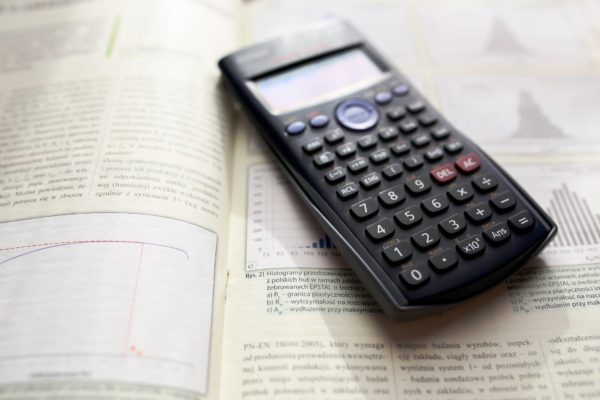# ACT Math: A Calculated Analysis

By  Layton Funk

Published on  October 25, 2019# What Is This Section?

The ACT Math section contains 60 questions of increasing difficulty to complete in 60 minutes.  Subjects range from algebra to geometry to trigonometry with some other assorted topics (statistics, logarithms, data interpretation, etc.) occasionally tested on throughout.  The distribution of these subjects varies from test to test, but typically the questions will be about 50-60% algebra, 20-25% geometry, 10-15% trigonometry, and 5-10% other assorted topics.  Fortunately, you are allowed to use a calculator on this part of the exam – a graphing calculator, actually! The main challenges in the math section are remembering a multitude of equations that the exam will not give you as well as pacing yourself given the number of questions.

Combining basic math skills with efficient utilization of your graphing calculator will be more than important for this section as you are on a fairly restrictive time limit of one minute per question on average.  Some of the questions will take mere seconds while others may take minutes to complete. Since every question is weighted the same in terms of points, it is recommended to skip harder ones in favor of easy questions (for you) to maximize the points you will receive.  The best way to study for the math sections is to do as many math problems as possible and commit the equations that they don’t give you to memory.

# Math Skills

There are many abstract skills that grant us the ability to solve problems, especially involving numbers, that studying and practicing any math course may reinforce in us:

• Identifying and stating a problem
• Planning a course of action
• Understanding multiple ways to solve a problem
• Critical thinking
• Asking the right questions
• Quantitative reasoning
• Ability to manipulate precise and intricate ideas
• Recalling something complicated from memory
• Constructing logical arguments and exposing illogical arguments
• Communication
• Deductive and inductive reasoning
• Learning from mistakes
• Time management
• Organization
• Independence

# Why Are These Skills Important?

Math skills are much more useful in life than people give them credit for.

• Balancing finances
• Calculating the tip after a meal
• Following a baking recipe
• Keeping track of time
• Planning an event

Those are just a few tangible real-life math skills we take for granted.  Not only that, but some of the best and most high paying jobs in the world require actual math skills: pharmacist, doctor, lawyer, accountant, engineer, software jobs, and more.  Aside from calculating numbers and interpreting data, Mathematics still has a fair amount of reading (especially in those nationally beloved word problems), but for the most part the ACT Math section is all about identifying what the question is asking and then finding some equation or number or graphical data point to solve it.  ffac

# Types of Questions

• Linear functions and inequalities, solving them, and graphing them
• Systems of equations/inequalities, solving them, and graphing them
• Algebraic proportions and word problems
• Rational and radical equations
• Properties and rules of exponents
• Absolute value, direct and inverse variation
• Polynomial equations, factoring, conjugates
• Area, perimeter, volume of geometric shapes
• Area and circumference of a circle, equation and graphing a circle. Arc lengths/sectors
• Pythagorean theorem, triangles
• Coordinate geometry, slope, transformations, parallel/perpendicular lines
• Data interpretation: mean, median, mode, standard deviation, probability/statistics
• Logarithms

# General Exam Tips

• Utilize process of elimination
• Show your work, always!
• Read questions and their answers carefully and completely
• Determine the type of question at hand and if it combines multiple topics/methods
• Identify what the question is asking for – what form and units is the answer in?
• Find what your weakness is – mathematics skills or timing
• Start studying by taking Math section after Math section, timing yourself
• Get good at basic addition and subtraction as well as your multiplication tables (and perfect squares) – don’t use up time plugging numbers into the calculator
• Only use your calculator if you absolutely have to
• Double check your answers if you have time
• Commit important equations to memory – trig identities, volume/area, quadratic formula, data interpretation & statistics, etc.
• If you don’t know how to solve a problem, try working backwards from the answers. Only do this if these are the only questions left!
• Keep track of the time, but don’t rush! Nothing wastes more time than hurrying
• Don’t leave any questions blank
• If there is a question you’re not sure about, mark it and come back to it. No sense dwelling on a question for an overly long time
• Stay on top of bubbling – if you skip around, make sure you bubble the right questions!
• Practice, practice, practice